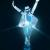## 求上下滚动，或者左右滚动的JS代码，越强大越好

PHPer_Dev 发布于 2011/11/02 20:31

00var Speed_1 = 10; //速度(毫秒)
var Space_1 = 24; //每次移动(px)
var PageWidth_1 = 128 * 6; //翻页宽度
var interval_1 = 6000; //翻页间隔时间
var fill_1 = ; //整体移位
var MoveLock_1 = false;
var MoveTimeObj_1;
var MoveWay_1="right";
var Comp_1 = ;
var AutoPlayObj_1=null;
function GetObj(objName){if(document.getElementById){return eval('document.getElementById("'+objName+'")')}else{return eval('document.all.'+objName)}}
function AutoPlay_1(){clearInterval(AutoPlayObj_1);AutoPlayObj_1=setInterval('ISL_GoDown_1();ISL_StopDown_1();',interval_1)}
function ISL_GoUp_1(){if(MoveLock_1)return;clearInterval(AutoPlayObj_1);MoveLock_1=true;MoveWay_1="left";MoveTimeObj_1=setInterval('ISL_ScrUp_1();',Speed_1);}
function ISL_StopUp_1(){if(MoveWay_1 == "right"){return};clearInterval(MoveTimeObj_1);if((GetObj('ISL_Cont_1').scrollLeft-fill_1)%PageWidth_1!=){Comp_1=fill_1-(GetObj('ISL_Cont_1').scrollLeft%PageWidth_1);CompScr_1()}else{MoveLock_1=false}
AutoPlay_1()}
function ISL_ScrUp_1(){if(GetObj('ISL_Cont_1').scrollLeft<=){GetObj('ISL_Cont_1').scrollLeft=GetObj('ISL_Cont_1').scrollLeft+GetObj('List1_1').offsetWidth}
GetObj('ISL_Cont_1').scrollLeft-=Space_1}
function ISL_GoDown_1(){clearInterval(MoveTimeObj_1);if(MoveLock_1)return;clearInterval(AutoPlayObj_1);MoveLock_1=true;MoveWay_1="right";ISL_ScrDown_1();MoveTimeObj_1=setInterval('ISL_ScrDown_1()',Speed_1)}
function ISL_StopDown_1(){if(MoveWay_1 == "left"){return};clearInterval(MoveTimeObj_1);if(GetObj('ISL_Cont_1').scrollLeft%PageWidth_1-(fill_1>=?fill_1:fill_1+1)!=){Comp_1=PageWidth_1-GetObj('ISL_Cont_1').scrollLeft%PageWidth_1+fill_1;CompScr_1()}else{MoveLock_1=false}
AutoPlay_1()}
function ISL_ScrDown_1(){if(GetObj('ISL_Cont_1').scrollLeft>=GetObj('List1_1').scrollWidth){GetObj('ISL_Cont_1').scrollLeft=GetObj('ISL_Cont_1').scrollLeft-GetObj('List1_1').scrollWidth}
GetObj('ISL_Cont_1').scrollLeft+=Space_1}
function CompScr_1(){if(Comp_1==){MoveLock_1=false;return}
var num,TempSpeed=Speed_1,TempSpace=Space_1;if(Math.abs(Comp_1)<PageWidth_1/2){TempSpace=Math.round(Math.abs(Comp_1/Space_1));if(TempSpace<1){TempSpace=1}}
if(Comp_1<){if(Comp_1<-TempSpace){Comp_1+=TempSpace;num=TempSpace}else{num=-Comp_1;Comp_1=}
GetObj('ISL_Cont_1').scrollLeft-=num;setTimeout('CompScr_1()',TempSpeed)}else{if(Comp_1>TempSpace){Comp_1-=TempSpace;num=TempSpace}else{num=Comp_1;Comp_1=}
GetObj('ISL_Cont_1').scrollLeft+=num;setTimeout('CompScr_1()',TempSpeed)}}
function picrun_ini(){
GetObj("List2_1").innerHTML=GetObj("List1_1").innerHTML;
GetObj('ISL_Cont_1').scrollLeft=fill_1>=?fill_1:GetObj('List1_1').scrollWidth-Math.abs(fill_1);
GetObj("ISL_Cont_1").onmouseover=function(){clearInterval(AutoPlayObj_1)}
GetObj("ISL_Cont_1").onmouseout=function(){AutoPlay_1()}
AutoPlay_1();
}
</script>